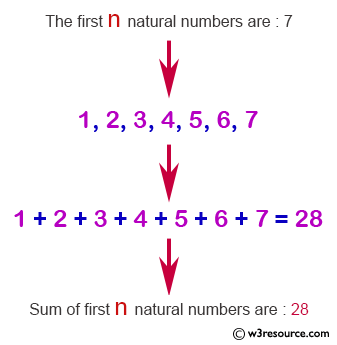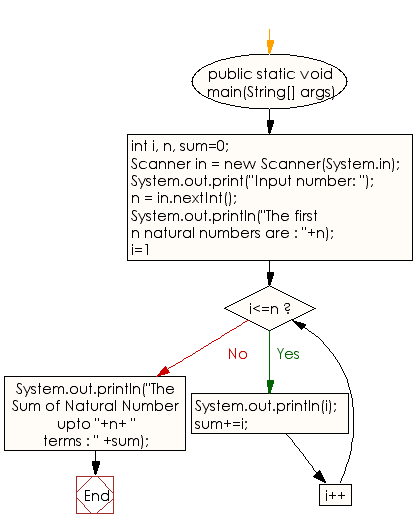﻿ Java exercises: Display n terms of natural numbers and their sum - w3resource# Java Conditional Statement Exercises: Display n terms of natural numbers and their sum

## Java Conditional Statement: Exercise-11 with Solution

Write a program in Java to display n terms of natural numbers and their sum.

Test Data
Input number: 7

Pictorial Presentation:Sample Solution:

Java Code:

``````import java.util.Scanner;
public class Exercise11 {

public static void main(String[] args)

{
int i, n, sum=0;
{
Scanner in = new Scanner(System.in);
System.out.print("Input number: ");
n = in.nextInt();
}
System.out.println("The first n natural numbers are : "+n);

for(i=1;i<=n;i++)
{
System.out.println(i);
sum+=i;
}
System.out.println("The Sum of Natural Number upto "+n+ " terms : " +sum);

}
}
```
```

Sample Output:

```Input number: 7
The first n natural numbers are : 7
1
2
3
4
5
6
7
The Sum of Natural Number upto n terms : 28
```

Flowchart:Java Code Editor: